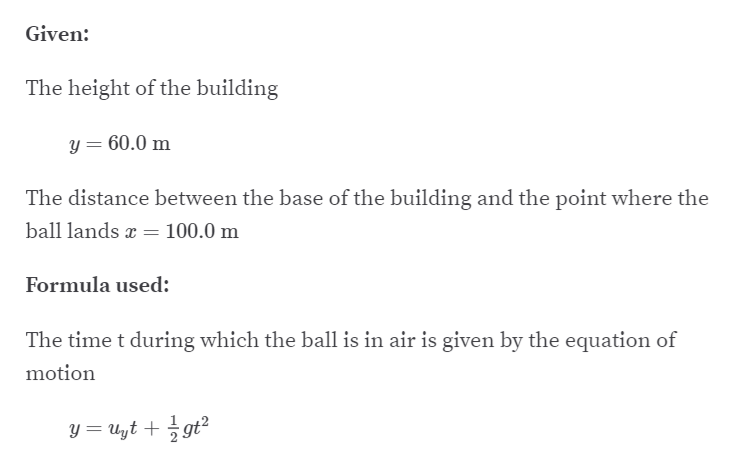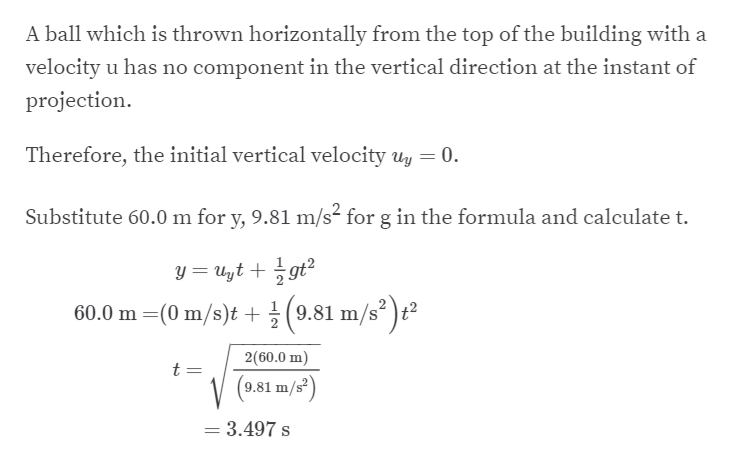# A ball is thrown horizontally from the top of a 60.0 m building and lands 100.0 m from the base of the building. How long is the ball in the air?

Question
11 views

A ball is thrown horizontally from the top of a 60.0 m building and lands 100.0 m from the base of the building. How long is the ball in the air?

check_circle

Step 1

Here,help_outlineImage TranscriptioncloseGiven: The height of the building y = 60.0 m The distance between the base of the building and the point where the ball lands a = 100.0 m Formula used: The time t during which the ball is in air is given by the equation of motion y = Uyt + gt? fullscreen
Step 2

Thus,&nbs...help_outlineImage TranscriptioncloseA ball which is thrown horizontally from the top of the building with a velocity u has no component in the vertical direction at the instant of projection. Therefore, the initial vertical velocity uy = 0. Substitute 60.0 m for y, 9.81 m/s² for g in the formula and calculate t. y = Uyt + gt? 60.0 m =(0 m/s)t + (9.81 m/s² )t² 2(60.0 m) t = 9.81 m = 3.497 s fullscreen

### Want to see the full answer?

See Solution

#### Want to see this answer and more?

Solutions are written by subject experts who are available 24/7. Questions are typically answered within 1 hour.*

See Solution
*Response times may vary by subject and question.
Tagged in

### Other